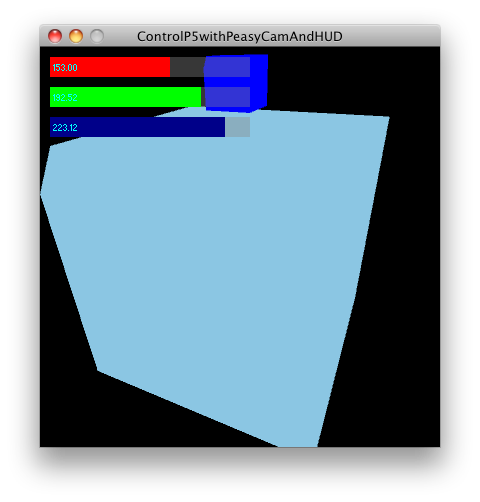﻿ Codec ░ Processing

## 737Processing Math convenience methods in pure Javascript

Like everybody else, I am huge fan of Processing, and like everybody else I find myself working more and more with Javascript. There is a implementation port of Processing (aptly called ProcessingJS) by John Resig of jQuery fame. It’s still lacking the 3D features, but not because they would be hard to implement in JS, but rather the OpenGL bindings in the browsers don’t exists wide-spreadly (yet). Let’s hope WebGL is going to change that soon. After working a lot with Processing, one becomes quite spoilt with it’s methods. The two best kept secrets of Processing are on the one hand the matrix state operations – pushMatrix(), and popMatrix() – and on the other hand the very handy map() method, basically mapping a number from one range to another. In Javascript’s canvas you have the pushMatrix() popMatrix() equivalents as save() and restore(). The map() methods does not exists as such, but it is easy implemented. I took a look at the Processing source and rewrote the Java methods in Javascript.
```var p5 = {};

p5.sq = function(a) {
return a*a;
}

p5.constrain = function(amt, low, high) {
return (amt < low) ? low : ((amt > high) ? high : amt);
}

}

p5.mag = function(a, b) {
return Math.sqrt(a*a + b*b);
}

p5.dist = function(x1, y1, x2, y2) {
return Math.sqrt(p5.sq(x2-x1) + p5.sq(y2-y1));
}

p5.lerp = function(start, stop, amt) {
return start + (stop-start) * amt;
}

p5.norm = function(value, start, stop) {
return (value - start) / (stop - start);
}

p5.map = function (value, istart, istop, ostart, ostop) {
return ostart + (ostop - ostart) * ((value - istart) / (istop - istart));
}
```
The code including comments and a minified version is also up on GitHub, show you love and fork it. https://github.com/trembl/p5.Math.js/
Update – Extending Math
Rather than creating a dedicated object, and as all the function are kind of related to Math, I thought it would be a good idea to extend the Math object itself. (Well, it’s a good idea for my project – your mileage, especially if you are flying with a lot of libraries might vary). And how to you extend a Javascript build-in object? With prototype, right?
```Math.prototpe.sq = function(a) {
return a*a;
}
```
Wrong. Because Math does not have a constructor, it therefore hasn’t been constructed and therefore does not have the prototype method. It has been instantiated and you add methods (or functions?) like this:
```Math.sq = function(a) {
return a*a;
}
```
You get the idea. The new code also lives at GitHub, check it out. https://github.com/trembl/p5.Math.js/

## 673Checking mouseOver in controlP5

In sojamo’s controlP5 library for Processing, check globally, whether the mouse is over a controlP5 element. Particularly usefully when the mouseDrag is also mapped to something else. ``` controlP5.window(this).isMouseOver(); ``` sojamo’s original comment:

## 650Enabling Font Smoothing in the Processing IDE

Open your preferences.txt, set editor.antialias=true. Works with Processing 1.2.1

## 614ControlP5, PeasyCam and HUD in ProcessingA slight development of an ControlP5 example. I needed to have the controls over the PeasyCam, wasn’t too hard to achieve. Nice one, Processing. Here’s the applet.
```/**
* ControlP5 with PeasyCam support.
* Tested with Processing 1.2.1, PeasyCam 0.8.3 and ControlP5 0.5.0
*
* original by
* by jeffg 2009
* http://processing.org/discourse/yabb2/YaBB.pl?num=1234988194/30#30
*
* modified by trembl 2010
*/

import peasy.*;
import controlP5.*;
import processing.opengl.*;

PeasyCam cam;
ControlP5 controlP5;
PMatrix3D currCameraMatrix;
PGraphics3D g3;

int buttonValue = 1;

int myColor = color(255,0,0);
Slider r,gr,b;
void setup() {
size(400,400,OPENGL);
g3 = (PGraphics3D)g;
cam = new PeasyCam(this, 100);
cam.setMinimumDistance(-100);
cam.setMaximumDistance(200);

controlP5 = new ControlP5(this);

r.setColorActive(color(128,0,0));
r.setColorBackground( color(127,100) );
r.setColorForeground(color(255,0,0));
r.setColorLabel(color(0,255,255,127));
r.setColorValue(color(0,255,255,127));
r.setLabel("");
r.setLabelVisible(true);

gr.setColorActive(color(0,128,0));
gr.setColorBackground( color(127,100) );
gr.setColorForeground(color(0,255,0));
gr.setColorLabel(color(0,255,255,127));
gr.setColorValue(color(0,255,255,127));
gr.setLabel("");
gr.setLabelVisible(true);

b.setColorActive(color(0,0,128));
b.setColorBackground( color(127,100) );
b.setColorForeground(color(0,0,128));
b.setColorLabel(color(0,255,255,127));
b.setColorValue(color(0,255,255,127));
b.setLabel("");
b.setLabelVisible(true);

controlP5.setAutoDraw(false);
}
void draw() {

background(0);
fill(myColor);
box(30);
pushMatrix();
translate(0,0,20);
fill(0,0,255);
box(5);
popMatrix();

cam.beginHUD();
gui();
cam.endHUD();

cam.setMouseControlled(true);
if(r.isInside() || gr.isInside() || b.isInside() ) {
cam.setMouseControlled(false);
}
}

void gui() {
currCameraMatrix = new PMatrix3D(g3.camera);
camera();
controlP5.draw();
g3.camera = currCameraMatrix;
}

void redSlider(float v) {
myColor = color( v, green(myColor), blue(myColor) );
r.setColorActive(color(v,0,0));
}

void greenSlider(float v) {
myColor = color(red(myColor), v, blue(myColor) );
gr.setColorActive(color(0,v,0));
}

void blueSlider(float v) {
myColor = color(red(myColor), green(myColor), v );
b.setColorActive(color(0,0,v));
}
```
﻿